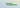# Converting decimal numbers to (binary,hexadecimal,octal) in JavaScriptIn this tutorial, we are going to learn about how to convert decimal numbers to a binary, hexadecimal and octal in JavaScript.

## Number.toString

In JavaScript, we have a `toString()` method which takes the base as an argument and returns the string of the specified base.

Let’s see the examples.

## Converting decimal to binary

To convert a decimal number to binary we need to pass `2` as an argument to `toString()` method because a binary number is expressed in base-2.

``````const num = 12;
//base 2 binary
console.log(num.toString(2));

//output --> "1100"``````

To convert a decimal number to hexadecimal we need to pass `16` as an argument to `toString()` method because a hexadecimal number is expressed in base-16.

``````const num = 12;
//base16 hex
console.log(num.toString(2));

//output --> "c"``````

## Converting decimal to octal

To convert a decimal number to octal we need to pass `8` as an argument to `toString()` method because an octal number is expressed in base-8.

``````const num = 12;
//base8 octal
console.log(num.toString(2));

//output --> "14"``````

## Top Udemy Courses##### JavaScript - The Complete Guide 2020 (Beginner + Advanced)
45,614 students enrolled
52 hours of video content
View Course##### React - The Complete Guide (incl Hooks, React Router, Redux)
284,472 students enrolled
40 hours of video content
View Course##### Vue JS 2 - The Complete Guide (incl. Vue Router & Vuex)
130,921 students enrolled
21 hours of video content
View Course